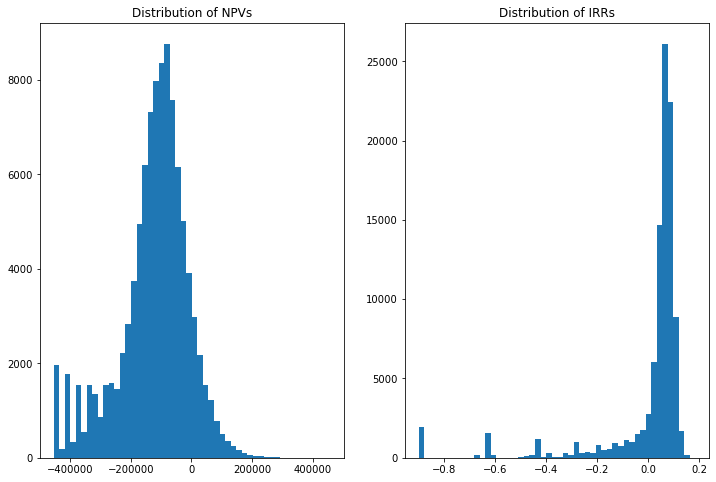# Monte Carlo simulations¶

This notebook computes expected cash-flows via Monte Carlo simulations in the context of a simple example. Specifically, it solves problem 2 at the end of chapter two of my notes. The problem considers a project that costs 500,000 to invest in today, yields 50k in year 1 and then random cash-flows for up to 20 years. The growth rate of cash-flows from year to year is drawn from a discrete dsitribution with four possible values. We will use slightly different parameters so as not to quite give the answer away.

As I say in class, Monte Carlo are an overkill here, we could solve this problem with pencil and paper -- just about. The point, as always, is to illustrate techniques that would become necessary in more complex versions of the problem.

Let's start by setting parameters.

In :
# Parameters

grovalue = [0,0.8,1,1.2] # possible growth rates (different values from those in my notes, on purpose)
probabilities = [0.02,0.1,0.78,0.1] # associated probabilities
rate=0.1 # discount rate
cost= 500000 # investment cost at date 0
cf1= 50000 # year 1 cash flow
life= 20 # max life of project

n=100000 # length of simulations


Now we invest in the project n times using numpy's random number generator

In :
import numpy as np
import pandas as pd

# invest in the projet n times
# the following line uses the power of Python list cmomprehension

tableofgrowth=np.array([np.random.choice(grovalue, 19, p=probabilities) for i in range(n)])
cashflows= cf1*np.ones((n,life))

# double loop to fill up cash flows

for i in range(n):
for j in range(1,life):
cashflows[i,j]=cashflows[i,j-1]*tableofgrowth[i,j-1]

# Now let's look at the first few histories of cash-flows

print(cashflows[0:5,:])

[[ 50000.    50000.    50000.    50000.    60000.    72000.    72000.
86400.    86400.    86400.    86400.    86400.    86400.   103680.
103680.   103680.   103680.   103680.   103680.    82944.  ]
[ 50000.    40000.    40000.    32000.    32000.    32000.    38400.
38400.    38400.    38400.    38400.    38400.    38400.    38400.
38400.    38400.    38400.    38400.    30720.    30720.  ]
[ 50000.    50000.    50000.    50000.    50000.    50000.    40000.
40000.    40000.    40000.    40000.    40000.    40000.    40000.
40000.    32000.    32000.    32000.    32000.    32000.  ]
[ 50000.    60000.    60000.    60000.    48000.    48000.    48000.
48000.    48000.    48000.    48000.    38400.    38400.    38400.
46080.    55296.    55296.    44236.8   44236.8   44236.8 ]
[ 50000.    40000.    40000.    40000.    32000.    25600.    25600.
25600.    30720.    30720.    36864.    36864.    36864.    36864.
44236.8   44236.8   44236.8   35389.44  35389.44  35389.44]]


Let us compute and plot the NPV and IRR of each history. Formally, speaking of "distributions of NPVs" or "distributions of IRRs" is an abuse of language. There is only one IRR and one NPV, those associated with the one and only expected cash-flow paths. The abuse of language is common and mostly harmless so we will do it too but that puts us one step closer to speaking of highly misleading objects such as the average IRR, which has zero meaning in Finance.

In :
rate= 0.1;
import numpy_financial as npf

# Python list comprehension syntax is beautiful
# The following adds the initial cost to all cash flow histories and then computes npv and irr
# history by history, in one line

results=[[npf.npv(rate, row).round(2),npf.irr(row)]
for row in [np.concatenate(([-cost],cashflows[j,:]),axis=None) for j in range(n)]]

npv=[item for item in results]
irr=[item for item in results]

import matplotlib.pyplot as plt #graphing module with matlab-like properties
%matplotlib inline

fig, axs  = plt.subplots(1,2,figsize=(12, 8))

axs.hist(npv, bins=50)
axs.set_title("Distribution of NPVs")
axs.hist(irr, bins=50)
axs.set_title("Distribution of IRRs")

Out:
Text(0.5, 1.0, 'Distribution of IRRs')Those spikes on the left-hand side of both histograms may look strange at first glance. Either we did something wrong or, on the contrary, should we be able to explain them, we have evidence that we did things right. Either way, this is a great way to test our code.

Take the leftmost spike. It has to be the worst NPV history, which is the case in which the project fully collapses after the first cash-flow of 50k in year 1. Given our specified distribution this occurs 2% of the time hence, we'd expect, around 2,000 times out of 100,000 histories and that's exactly what we're getting. The other spikes to the right of that one are for cases where the project collapses after 3 years, then 4 years, and so on. Then the spikes start blending in with the rest of less calamitous histories. So it all makes sense, we must have done this right.

Now we compute the one and only NPV and the one and only IRR for this project. The key is to compute expected cash-flows first and then apply NPV and IRR to that one and only history.

In :
# First, always first, compute expected cash-flows

Ecf=np.mean(cashflows, axis=0) # the axis thingie says whether we're doing rows or columns. Here we want columns.

# now add the cost part

Ecf0=np.concatenate(([-cost],Ecf),axis=None)
npv0=npf.npv(rate, Ecf0)
irr0=100*npf.irr(Ecf0)

# And now we are done

print('The project\'s NPV is %.2f' %npv0)
print('The project\'s IRR is %.2f percent' %irr0)

The project's NPV is -124431.57
The project's IRR is 5.87 percent